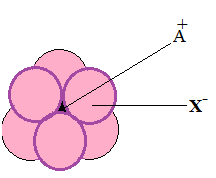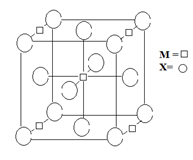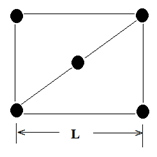×#### Thank you for registering.

One of our academic counsellors will contact you within 1 working day.

Click to Chat

1800-1023-196

+91-120-4616500

CART 0

• 0

MY CART (5)

Use Coupon: CART20 and get 20% off on all online Study Material

ITEM
DETAILS
MRP
DISCOUNT
FINAL PRICE
Total Price: Rs.

There are no items in this cart.
Continue Shopping• Complete JEE Main/Advanced Course and Test Series
• OFFERED PRICE: Rs. 15,900
• View Details

```Question: 1 The arrangement of X– ions around A+ ion in solid AX is given in the figure (not drawn to scale). If the radius of X–is 250 pm, the radius of A+ is (IIT JEE 2013)1. 104 pm

2. 125 pm

3. 183 pm

d. 57 pm

Solution:

A+ is present in the octahedral void of X-.

For octahedral Void

r+/r- = 0.414

or

r+ = 0.414 r- = 0.414 × 250 pm ≈104 pm

Hence, the correct option is a.Question:2 A compound MpXq has cubic close packing (ccp) arrangement of X. Its unit cell structure is shown below.(IIT JEE-2012)The empirical formula of the compound is

1. MX

2. MX2

3. M2X

4. M5X14

Solution:

X- ions are present at the corners and  face centers:

Number of x- ions per lattice = 1/8(Number of ions present at corners of cube) + ½(Number of ions present at face centers) = 1/8(8) + ½(6) = 4

M+ ions are present at the four edge centers and at body center. corners and on face centers:

Number of M+ ions per lattice = 1/4(Number of ions present at edge centers of cube) + (Number of ions present at body center) = 1/4(4) + 1 = 2

Now: X: M = 4:2 = 2:1

So, the formula should be MX2

Hence, the correct option is b.

Question:3 The packing efficiency of the two-dimensional square unit cell shown below is   (IIT JEE-2010)1) 39.27%

2) 68.02%

3) 74.05%

4) 78.54%

Solution:Total number of the atoms per unit cell = ¼(4) +1 = 2

Let, Radious of the atom = r

SR = QR =PQ = SP =L

&

QS = 4r

Now, in triangle SRP,

SR2 + QR2 =SQ2

Or

L2+L2 =(4r)2

r = L/(2√2 )

Or

Packing Efficiency = [(2× Volume of one atom)/(Total volume of the unit cell)]×100

=100 × {2π[L/(2√2 )]2 }/L2

= (π/8 )×100 = 78.54%

Hence, the correct option is D.
```### Course Features

• 728 Video Lectures
• Revision Notes
• Previous Year Papers
• Mind Map
• Study Planner
• NCERT Solutions
• Discussion Forum
• Test paper with Video Solution